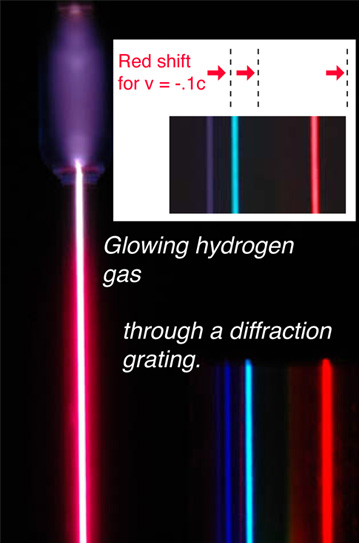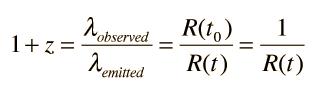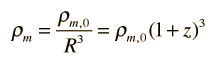# Red Shift

The light from distant stars and more distant galaxies is not featureless, but has distinct spectral features characteristic of the atoms in the gases around the stars. When these spectra are examined, they are found to be shifted toward the red end of the spectrum. This shift is apparently a Doppler shift and indicates that essentially all of the galaxies are moving away from us. Using the results from the nearer ones, it becomes evident that the more distant galaxies are moving away from us faster. This is the kind of result one would expect for an expanding universe. The red line of the spectrum below is the transition from n=3 to n=2 of hydrogen and is famous as the H-alpha line seen throughout all the universe.Calculation
 Hydrogen spectrum

The building up of methods for measuring distance to stars and galaxies led Hubble to the fact that the red shift (recession speed) is proportional to distance. If this proportionality (called Hubble's Law) holds true, it can be used as a distance measuring tool itself.

The measured red shifts are usually stated in terms of a z parameter. The largest measured z values are associated with the quasars.Hydrogen redshift example
 Examples of observed redshifts Redshift calculation example
Index

 HyperPhysics***** Astrophysics R Nave
Go Back

# Measured Red Shifts

Measured Doppler red shifts give the recession velocity of stars or galaxies, presuming that the Hubble law is valid. It is common practice to express this velocity as a fraction of the speed of light and to use a parameter z defined by:For z = v/c =

where λ is understood to be λemitted = λrest, in the rest frame of the emitter.
 Calculating v/c from this expression gives:The largest red shifts measured are those of the quasars. Measurements of >100 quasars have given a range of 0.16 to 3.53, corresponding to recession speeds of 0.15c to 0.91c.

Another application of the z parameter is to imply the scale factor R of the universe at the time that light was emitted from a given observed object. The z parameter is related to R by the expressionwhere the scale is taken as R0 =1 at the present time. For example, if the redshift of an object is found to correspond to z=3, then R=1/4 and we imply that the universe has expanded by a factor of four since light left that object. The wavelength of the received radiation has expanded by a factor of four because space has expanded by a factor of four during its transit from the emitting object. At such large values of z, the redshift is mainly the cosmological redshift, and not a valid measure of the actual recessional velocity of the object with respect to us.

The z parameter can also be used to assess the mass density compared to the mass density ρ0 at the present time.With z=3, the density is 64 times that of the present density.

 Examples of observed redshifts Redshift calculation example
Index

Reference:
Carroll & Ostlie
Sec 4.3

 HyperPhysics***** Astrophysics R Nave
Go Back

# Red Shift of Galaxy 8C1435+635

Reported in November 1994 in Monthly Notices of the Royal Astronomical Society is a galaxy with a measured red shift of z=4.25 , a new record. This value for the z parameter corresponds to a recession speed of .93c. The galaxy 8C1435+635 was observed in a systematic search for faint, radio-emitting galaxies carried out by a team at Leiden Observatory led by George Miley. After discovery, the optical spectra was observed by the William Hershel Telescope in La Palma, Canary Islands. Two emission lines of ionized carbon and hydrogen were measured to obtain the red shift. This red shift corresponds to a distance of about 13 billion light years if one uses the current WMAP value of 71km/s/mpc for the Hubble parameter is used.

Index

 HyperPhysics***** Astrophysics R Nave
Go Back